Areas of Regular Polygons MathBitsNotebook.com Terms of Use   Contact Person: Donna RobertsRemember that "regular" polygons have all sides congruent and all angles congruent. Regular polygons have a center and a radius (coinciding with their circumscribed circle), and the distance from the center perpendicular to any side is called its apothem.

 Regular Pentagon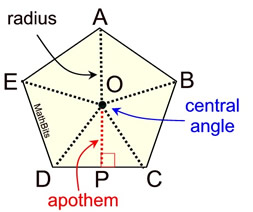The apothem of a regular polygon is a line segment from the center of the polygon perpendicular to any side of the polygon. Triangle DOC is an isosceles triangle, making the apothem the altitude of this triangle and the median of this triangle (going to the midpoint P.) The apothem is also the radius of the inscribed circle. The apothem can be used to determine area: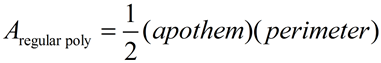Area of a REGULAR polygon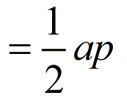(where a = apothem and p = perimeter)

Here are the more popular regular polygons.
(Remember: The formula for each interior angle of a regular polygon is [180(n - 2)] / n where n = the number of sides.)

 Equilateral Triangle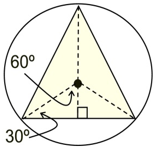interior angle = 60º Special 30º-60º-90º Δs at work! Square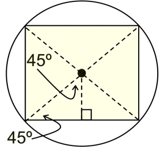interior angle = 90º Special 45º-45º-90º Δs at work! Regular Pentagon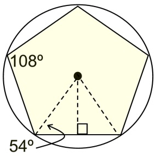interior angle = 108º Must use trig. to work in this triangle. Regular Hexagon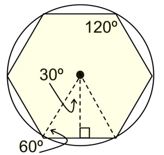interior angle = 120º Special 30º-60º-90º Δs at work! Regular Octagon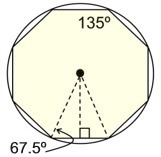interior angle = 135º Must use trig. to work in this triangle.Using the strategy of partition or dissection, the areas of these regular polygons can be found by adding together the areas of all of the congruent triangles formed by the central angles and each side of the polygon.
The number of such triangles = the number of sides of the polygon.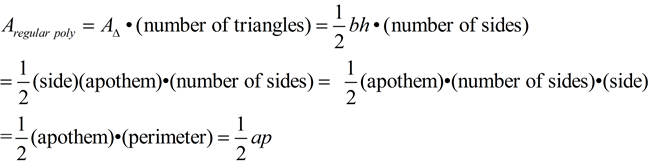In addition to using the strategy of partitioning or dissecting, it may also be possible to graph the polygon on a set of coordinate axes and determine the area using coordinate geometry techniques. A "grid" method may be useful if sufficient information is known.